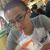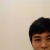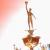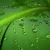# 补码之美

horst_hu 发布于 2014/01/22 11:06

`X - Y = X + (-Y)`

## 原码

1 和 0。于是，在计算机里，我们采用二进制来表示整数。

`1 - 1 = 1 + (-1) = 0001 + 1001 = 1010 = -2`

## 补码

```-1 = 0000 - 0001
= 10000 - 0001  （高位借一）
= (1111 + 0001) - 0001
= (1111 - 0001) + 0001（第一步：取反）
= 1110 + 0001（第二步：加一）
= 1111```

```-Y = 0000 - Y
= (1111 + 1 ) - Y
= (1111 - Y) + 1   将正整数 Y 的二进制先取反、再加一```

## 简单之美

1. 数轴上，左边的数永远小于右边的数。比如 -8 < -2 < 0 < 3 < 5
2. 数轴上，只有一个零。
3. 相邻整数之间相差一。

```-8      -7         -6        -5        -4        -3       -2      -1       0          1         2        3         4        5         6         7
1000 | 1001 |  1010 | 1011 | 1100 | 1101 | 1110 | 1111 | 0000 | 0001 | 0010 | 0011 | 0100 | 0101 | 0110 | 0111```
1. 只看负数的补码表示，明显 1000 < 1001，补码表示下，左边的负数很自然小于右边的负数。
2. 很明显只有一个零，就是 0000，不存在 -0 跟 0 的表示不一样。
3. 再看相邻整数之间，左边的整数加一，就是右边的整数，包括 1111 + 1 = 0000 。

（完）

000`= 10000 - 0001  （高位借一）`@翟志军 你要想想它前面还有一位就想了，没有超过计算机的寻址空间就行了0#### 引用来自“gzwxn”的答案